Finding Potential Difference Between Two Points in Circuits with Examples

Finding the Potential Difference between the Two Points in Circuits

Potential difference between two points in circuit is the energy lost by the charge in being transferred from one point to another. For example, potential difference between A and B is found with following formula;

VAB=VB-VA=∑ε-∑i.R

This formula shows the energy lost by charge moving from point A to point B.

We should first find the direction of the current to determine values ∑ε and ∑i.R. If you take the sign of batteries having same direction with current as “+” then you must take oppositely connected batteries as “-“.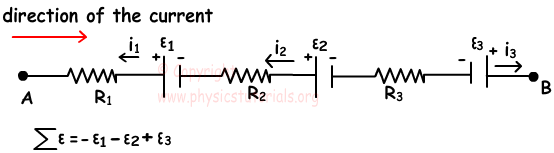If the direction of the current and current passing through the resistor are the same then we take i.R as "+", if they are in opposite directions then we take i.R as "-".

In the circuit given below, if the direction of the current is shown like below, then potential difference between the points A-B and C-B are;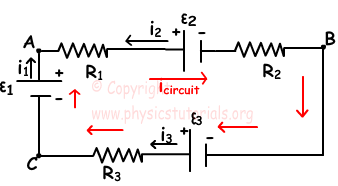VAB=VB-VA= -ε2-(+i.R1+i.R2)

VCB=VB-VC= -ε3-(-i.R3)

Example: Find the potential difference between the points A and B in the given circuit below.

Current of the circuit is in the direction of battery current.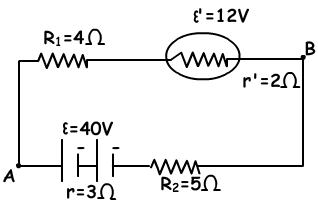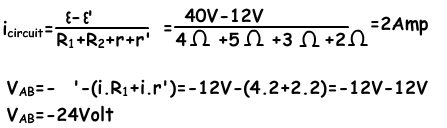Example: Find the power of the resistor R1.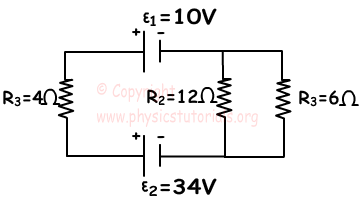εeq21=34V-10V=24V

Equivalent resistance of the circuit is;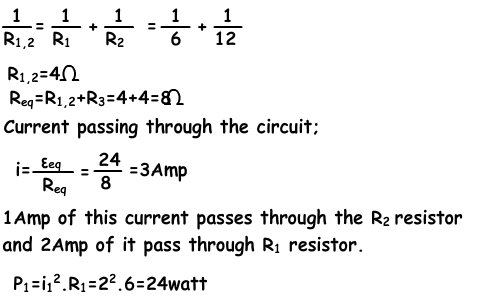Electric Current Exams and Solutions

Author: